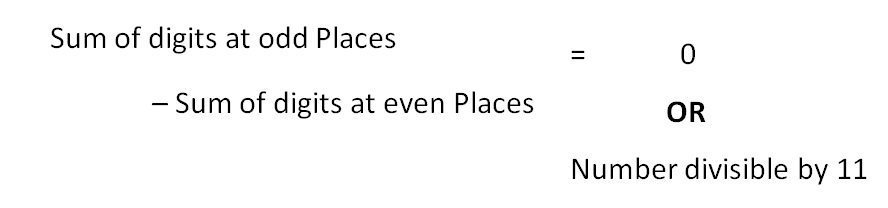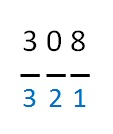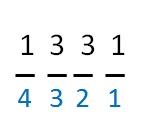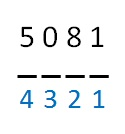Divisibility Tests - Divisibility by 11

Chapter 3 Class 6 Playing with Numbers
Concept wise

IfSo, if the difference is 0, 11, 22, 33, ….

Then, Number is divisible by 11

## Is 308 divisible by 11?Sum of digits at odd Places = 8 + 3 = 11

Sum of digits at even Places = 0

Difference = 11 − 0 = 11

Since 11 is divisible by 11

∴ 308 is divisible by 11

## Is 1331 divisible by 11?Sum of digits at odd Places = 1 + 3 = 4

Sum of digits at even Places = 3 + 1 = 4

Difference = 4 − 4 = 0

Since difference is 0

∴ 1331 is divisible by 11

## Is 61809 divisible by 11?Sum of digits at odd Places = 9 + 8 + 6 = 23

Sum of digits at even Places = 0 + 1 = 1

Difference = 23 − 1 = 22

Since 22 is divisible by 11

∴ 61809 is divisible by 11

## Is 5081 divisible by 11?Sum of digits at odd Places = 1 + 0 = 1

Sum of digits at even Places = 8 + 5 = 13

Difference = 13 − 1 = 12

Since 12 is not divisible by 11

∴ 5081 is not divisible by 11

Get live Maths 1-on-1 Classs - Class 6 to 12

### Transcript

Sum of digits at odd Places – Sum of digits at even Places = 0 OR Number divisible by 11